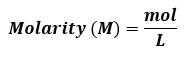# Problem: The total concentration of dissolved particles in blood is 0.30 M. An intravenous (IV) solution must be isotonic with blood, which means it must have the same concentration.(c) If the patient is given 150. mL/h of IV saline for 1.5 h, how many grams of NaCl did she receive?

###### FREE Expert Solution

Recall the molarity:Given information:

IV Saline solution → NaCl solution

Concentration of dissolved particles in blood = concentration of NaCl solution

[NaCl] = 0.30 M = 0.30 mol/L

94% (135 ratings)###### Problem Details

The total concentration of dissolved particles in blood is 0.30 M. An intravenous (IV) solution must be isotonic with blood, which means it must have the same concentration.

(c) If the patient is given 150. mL/h of IV saline for 1.5 h, how many grams of NaCl did she receive?